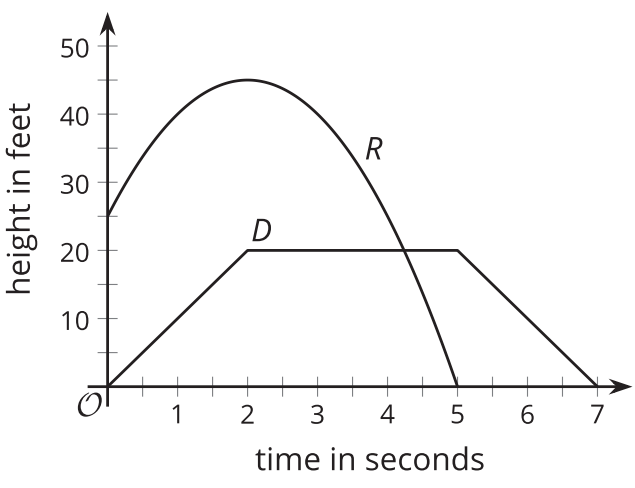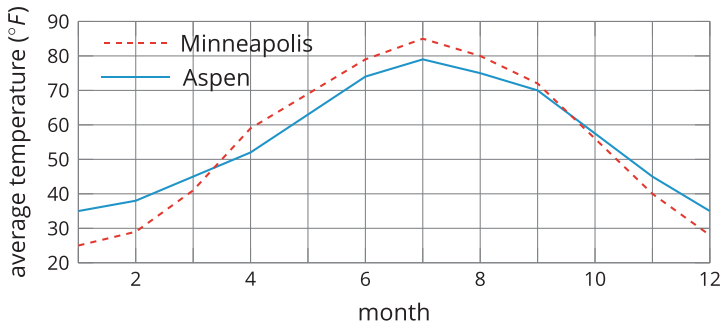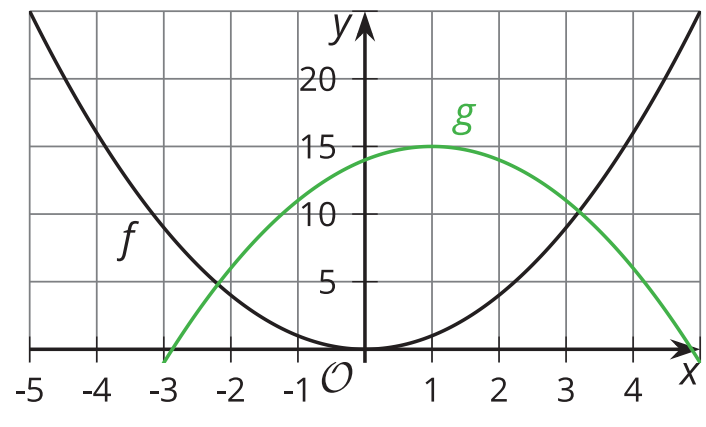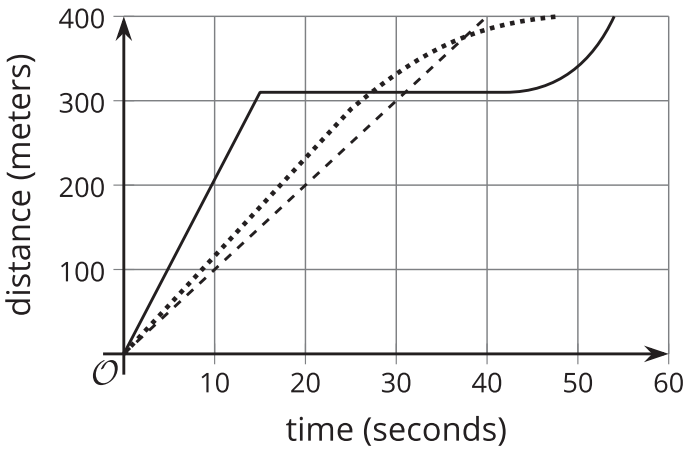# A.4.9 Practice Problems

## Problem 1

Functions R and D give the height, in feet, of a toy rocket and a drone, t seconds after they are released. Here are the graphs of R (for the rocket) and D (for the drone).Write each statement in function notation: a. At 3 seconds, the toy rocket is higher than the drone. b. At the start, the toy rocket is 25 feet above the drone.

## Problem 2

A(t) is the average high temperature in Aspen, Colorado, t months after the start of the year. M(t) is the average high temperature in Minneapolis, Minnesota, t months after the start of the year. Temperature is measured in degrees Fahrenheit.Which function had the higher average rate of change between the beginning of January and middle of March? What does this mean about the temperature in the two cities?

## Problem 3

Here are two graphs representing functions f and g.Select all statements that are true about functions f and g.

Check all that apply

## Problem 4

The three graphs represent the progress of three runners in a 400-meter race. The solid line represents runner A. The dotted line represents runner B. The dashed line represents runner C.a. One runner ran at a constant rate throughout the race. Which one? b. A second runner stopped running for a while. Which one? During which interval of time did that happen? c. Describe the third runner's race. Be as specific as possible. d. Who won the race? Explain how you know.

## Problem 5

Function A gives the area, in square inches, of a square with side length x inches.

## Problem 6

Function f is represented by f(x)=5(x+11). a. Find f(-2). b. Find the value of x such that f(x)=90 is true.